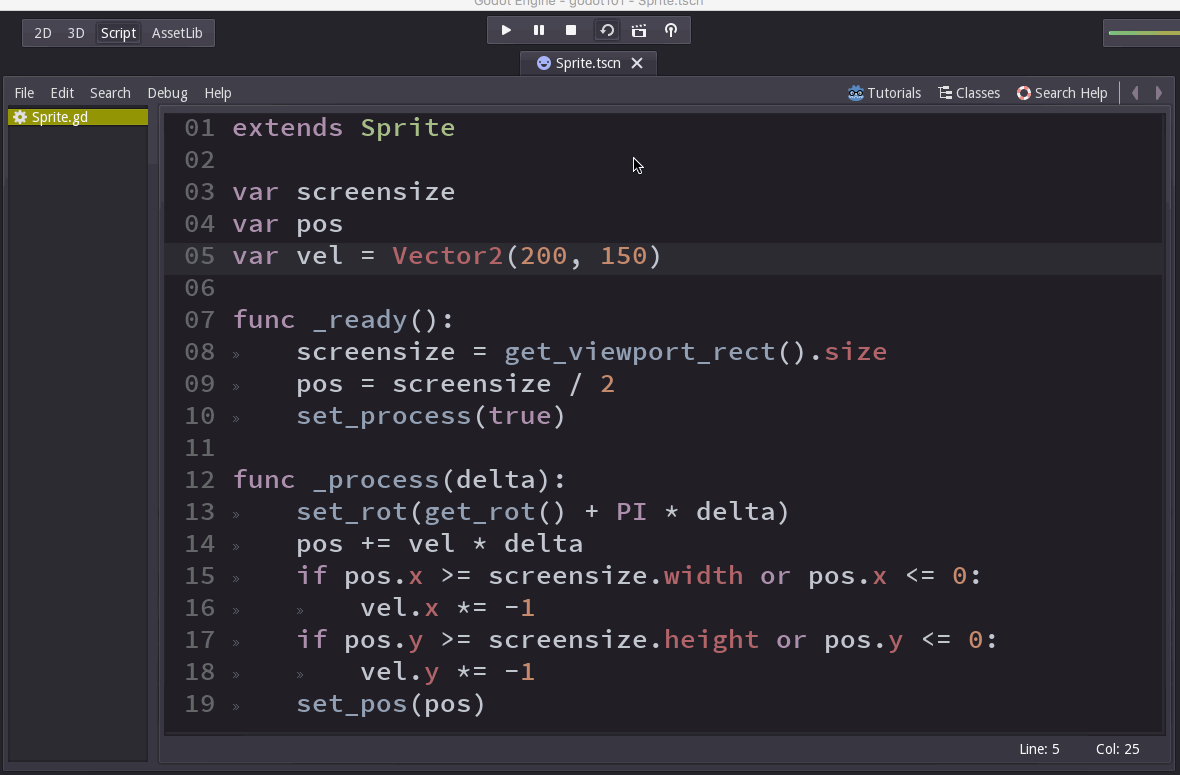# Godot 101 - Part 3: Scripting (continued)

###### Tags: godottutorialgamedevThis is part 3 of “Godot 101”, where we’ll finish scripting our bouncing animated sprite. If you haven’t already read through the previous parts, please start with Part 1.

Godot 101 is an introduction to the Godot game engine and how it works. If you’ve never used a game engine before, or if you’re just new to Godot, this is the place to start. If you’re new here, a quick note about this website: we’re called KidsCanCode because we teach programming and game development to kids, but if you’re an adult you’re welcome here, too. We don’t believe in dumbing-down the material for kids, and game development is hard - so it will probably be challenging no matter what your age.

You can watch a video version of this lesson here:

## Scripting (continued)

In the last lesson, we created a script to move our `Sprite`, but it goes off the edge of the screen, and we want to change that. We’re going to do it in a simple way first, then modify it to be more robust.

First, we need to know how big our screen is. Screen size is set in the Project Settings (click Scene -> Project Settings), but we need our script to use those values:

``````extends Sprite

var screensize
var pos
var vel = Vector2(100, 50)

screensize = get_viewport_rect().size
pos = screensize / 2
set_process(true)

func _process(delta):
set_rot(get_rot() + PI * delta)
pos += vel * delta
if pos.x >= screensize.width or pos.x <= 0:
vel.x *= -1
if pos.y >= screensize.height or pos.y <= 0:
vel.y *= -1
set_pos(pos)
``````

`get_viewport_rect()` gives us the screensize (you can look it up in the help if you want to see the details - or click here to see the docs).

Then we use a new variable, `pos`, to keep track of our position. If its `x` or `y` goes out of bounds, we reverse the velocity in that axis. Now we stay on the screen, but we still have a problem:Click to enlarge

The sprite doesn’t bounce until it goes halfway off the screen. That’s because the `Pos` property is the center of the sprite (there’s a checkbox marked `Centered` that will change it to the upper left). That means we need to calculate half the width & height of the sprite to have it bounce properly.

``````extends Sprite

var screensize
var extents
var pos
var vel = Vector2(200, 150)

screensize = get_viewport_rect().size
extents = get_texture().get_size() / 2
pos = screensize / 2
set_process(true)

func _process(delta):
set_rot(get_rot() + PI * delta)
pos += vel * delta
if pos.x >= screensize.width - extents.width:
pos.x = screensize.width - extents.width
vel.x *= -1
if pos.x <= extents.width:
pos.x = extents.width
vel.x *= -1
if pos.y >= screensize.height - extents.height:
pos.y = screensize.height - extents.height
vel.y *= -1
if pos.y <= extents.height:
pos.y = extents.height
vel.y *= -1
set_pos(pos)``````

We’re handling this with a new variable called `extents` which we fill using `get_texture()` - we also divide it by 2 because we only care about half of the width/height.

Next, we have to update our bouncing tests to add/subtract the extents to the wall positions, and now we’re bouncing correctly.

## Randomizing

As a last step, we’re going to randomize the velocity and spin using the `rand_range()` function:

``````extends Sprite

var screensize
var extents
var pos
var vel
var spin

randomize()
screensize = get_viewport_rect().size
extents = get_texture().get_size() / 2
pos = screensize / 2
vel = Vector2(rand_range(100, 300), 0).rotated(rand_range(0, 2*PI))
spin = rand_range(-PI, PI)
set_process(true)

func _process(delta):
set_rot(get_rot() + spin * delta)
pos += vel * delta
if pos.x >= screensize.width - extents.width:
pos.x = screensize.width - extents.width
vel.x *= -1
if pos.x <= extents.width:
pos.x = extents.width
vel.x *= -1
if pos.y >= screensize.height - extents.height:
pos.y = screensize.height - extents.height
vel.y *= -1
if pos.y <= extents.height:
pos.y = extents.height
vel.y *= -1
set_pos(pos)``````

One important thing to keep in mind when using randomized commands: by default, Godot will produce the same sequence of “random” numbers every time you run the game unless you first use `randomize()`.

## Wrapping up

That completes our bouncing sprite. In the next lesson, we’ll explore how to use this Sprite scene to add to a larger scene and allow us to spawn multiple sprites.